Q-Chem 4.3 User’s Manual

# 10.12 Vibrational Analysis

Vibrational analysis is an extremely important tool for the quantum chemist, supplying a molecular fingerprint which is invaluable for aiding identification of molecular species in many experimental studies. Q-Chem includes a vibrational analysis package that can calculate vibrational frequencies and their Raman  and infrared activities. Vibrational frequencies are calculated by either using an analytic Hessian (if available; see Table 9.1) or, numerical finite difference of the gradient. The default setting in Q-Chem is to use the highest analytical derivative order available for the requested theoretical method.

When calculating analytic frequencies at the HF and DFT levels of theory, the coupled-perturbed SCF equations must be solved. This is the most time-consuming step in the calculation, and also consumes the most memory. The amount of memory required is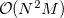where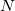is the number of basis functions, and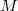the number of atoms. This is an order more memory than is required for the SCF calculation, and is often the limiting consideration when treating larger systems analytically. Q-Chem incorporates a new approach to this problem that avoids this memory bottleneck by solving the CPSCF equations in segments . Instead of solving for all the perturbations at once, they are divided into several segments, and the CPSCF is applied for one segment at a time, resulting in a memory scaling of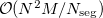, where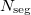is the number of segments. This option is invoked automatically by the program.

Following a vibrational analysis, Q-Chem computes useful statistical thermodynamic properties at standard temperature and pressure, including: zero-point vibration energy (ZPVE) and, translational, rotational and vibrational, entropies and enthalpies.

The performance of various ab initio theories in determining vibrational frequencies has been well documented; see Refs. Murray:1992,Scott:1996,Johnson:1993d.

## 10.12.1 Job Control

In order to carry out a frequency analysis users must at a minimum provide a molecule within the $molecule keyword and define an appropriate level of theory within the$rem keyword using the $rem variables EXCHANGE, CORRELATION (if required) (Chapter 4) and BASIS (Chapter 7). Since the default type of job (JOBTYPE) is a single point energy (SP) calculation, the JOBTYPE$rem variable must be set to FREQ.

It is very important to note that a vibrational frequency analysis must be performed at a stationary point on the potential surface that has been optimized at the same level of theory. Therefore a vibrational frequency analysis most naturally follows a geometry optimization in the same input deck, where the molecular geometry is obtained (see examples).

Users should also be aware that the quality of the quadrature grid used in DFT calculations is more important when calculating second derivatives. The default grid for some atoms has changed in Q-Chem 3.0 (see Section 4.3.13) and for this reason vibrational frequencies may vary slightly form previous versions. It is recommended that a grid larger than the default grid is used when performing frequency calculations.

The standard output from a frequency analysis includes the following.

• Vibrational frequencies.

• Raman and IR activities and intensities (requires $rem DORAMAN). • Atomic masses. • Zero-point vibrational energy. • Translational, rotational, and vibrational, entropies and enthalpies. Several other$rem variables are available that control the vibrational frequency analysis. In detail, they are:

DORAMAN
 Controls calculation of Raman intensities. Requires JOBTYPE to be set to FREQ

TYPE:
 LOGICAL

DEFAULT:
 FALSE

OPTIONS:
 FALSE Do not calculate Raman intensities. TRUE Do calculate Raman intensities.

RECOMMENDATION:
 None

VIBMAN_PRINT
 Controls level of extra print out for vibrational analysis.

TYPE:
 INTEGER

DEFAULT:
 1

OPTIONS:
 1 Standard full information print out. If VCI is TRUE, overtones and combination bands are also printed. 3 Level 1 plus vibrational frequencies in atomic units. 4 Level 3 plus mass-weighted Hessian matrix, projected mass-weighted Hessian matrix. 6 Level 4 plus vectors for translations and rotations projection matrix.

RECOMMENDATION:
 Use default.

CPSCF_NSEG
 Controls the number of segments used to calculate the CPSCF equations.

TYPE:
 INTEGER

DEFAULT:
 0

OPTIONS:
 0 Do not solve the CPSCF equations in segments.User-defined. Usesegments when solving the CPSCF equations.

RECOMMENDATION:
 Use default.

Example 10.222  An EDF1/6-31+G* optimization, followed by a vibrational analysis. Doing the vibrational analysis at a stationary point is necessary for the results to be valid.

$molecule O 1 C 1 co F 2 fc 1 fco H 2 hc 1 hcp 3 180.0 co = 1.2 fc = 1.4 hc = 1.0 fco = 120.0 hco = 120.0$end

$rem JOBTYPE opt METHOD edf1 BASIS 6-31+G*$end

@@@

$molecule read$end

$rem JOBTYPE freq METHOD edf1 BASIS 6-31+G*$end